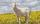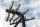# Equation + right triangle - examples

1. Find parametersFind parameters of the circle in the plane - coordinates of center and radius: ?
2. Trapezoid MOThe rectangular trapezoid ABCD with right angle at point B, |AC| = 12, |CD| = 8, diagonals are perpendicular to each other. Calculate the perimeter and area of ​​the trapezoid.
3. GardenArea of square garden is 6/4 of triangle garden with sides 56 m, 35 m and 35 m. How many meters of fencing need to fence a square garden?
4. Right ΔRight triangle has length of one leg 28 cm and length of the hypotenuse 53 cm. Calculate the height of the triangle.
5. Short cutImagine that you are going to the friend. That path has a length 330 meters. Then turn left and go another 2000 meters and you are at a friend's. The question is how much the journey will be shorter if you go direct across the field?
6. RiverFrom the observatory 14 m high and 32 m from the river bank, river width appears in the visual angle φ = 20°. Calculate width of the river.
7. Right triangle AlefThe obvod of a right triangle is 84 cm, the hypotenuse is 37 cm long. Determine the lengths of the legs.
8. Proof PTCan you easy prove Pythagoras theorem using Euclidean theorems? If so, do it.
9. RectangleIn rectangle ABCD with sides |AB|=19, |AD|=16 is from point A guided perpendicular to the diagonal BD, which intersects at point P. Determine the ratio ?.
10. R triangleCalculate the area of a right triangle whose longer leg is 6 dm shorter than the hypotenuse and 3 dm longer than the shorter leg.
11. MediansCalculate the sides of a right triangle if the length of the medians to the legs are ta = 21 cm and tb=12 cm.
12. ISO triangleCalculate the area of an isosceles triangle KLM if the length of its sides are in the ratio k:l:m = 4:4:3 and has perimeter 377 mm.
13. Leg and heightSolve right triangle with height v = 9.6 m and shorter cathetus b = 17.3 m.
14. Hypotenuse and heightIn a right triangle is length of the hypotenuse c = 56 cm and height hc = 4 cm. Determine the length of both trangle legs.
15. RT - hypotenuse and altitudeRight triangle BTG has hypotenuse g=117 m and altitude to g is 54 m. How long are hypotenuse segments?
16. RhombusInternal angles of rhombus is in ratio 2:3. How many times is the shorter diagonal longer than side of rhombus?
17. Nice prismCalculate the surface of the cuboid if the sum of its edges is a + b + c = 19 cm and the body diagonal size u = 13 cm.
18. GoatMeadow is a circle with radius r = 19 m. How long must a rope to tie a goat to the pin on the perimeter of the meadow to allow goat eat half of meadow?
19. ColumnPerpendicular pole high 8 m tall broke and its toe fell 2.7 m from the bottom of the pole. At what height above the ground pole broke?
20. TriangleCalculate the sides of the triangle if its area S = 630 and the second cathethus is shorter by 17.

Do you have an interesting mathematical example that you can't solve it? Enter it, and we can try to solve it.

To this e-mail address, we will reply solution; solved examples are also published here. Please enter e-mail correctly and check whether you don't have a full mailbox.

Do you have a linear equation or system of equations and looking for its solution? Or do you have quadratic equation? See also our right triangle calculator.